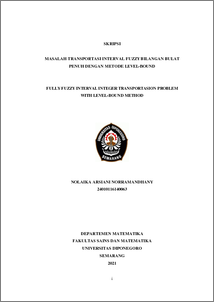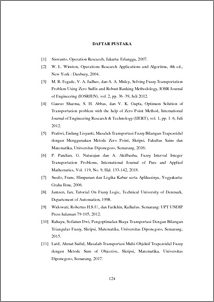# FULLY FUZZY INTERVAL INTEGER TRANSPORTASION PROBLEM WITH LEVEL-BOUND METHOD

Norramandhany, Nolaika Arsiani (2021) FULLY FUZZY INTERVAL INTEGER TRANSPORTASION PROBLEM WITH LEVEL-BOUND METHOD. Undergraduate thesis, UNDIP.Preview
PDF
194KbPDF
Restricted to Repository staff only

2095KbPreview
PDF
271Kb

## Abstract

Fully fuzzy interval integer transportation problem are transportation problem that supply, demand, and transportation costs are fuzzy interval integer, where in this discussion at each interval boundary using fuzzy numbers with triangular fuzzy numbers. This final project discusses the solution of fully fuzzy interval integer transportation problem by changing the fuzzy interval parameters into crisp numbers by using Level-Bound Methods, which are then solved by Least Cost Method, Modified Distribution Method and Zero Point Method. Furthermore, it is shown that the Level-Bound Method can provide an optimal solution to the minimum case of fully fuzzy interval integer transportation problem, both balanced and unbalanced. Keywords: Fully fuzzy interval integer transportation problem, fuzzy interval integer, Level-Bound Methods.

Item Type: Thesis (Undergraduate) Q Science > Q Science (General) Faculty of Science and Mathematics > Department of Mathematics 84166 INVALID USER 09 Jun 2022 14:03 09 Jun 2022 14:03

Repository Staff Only: item control page